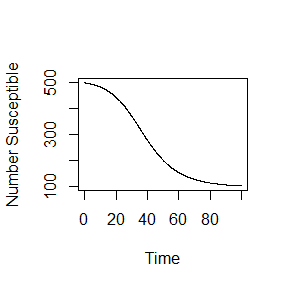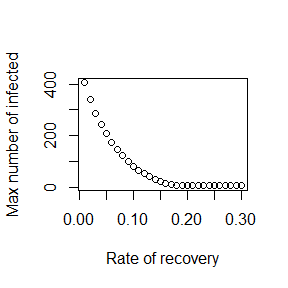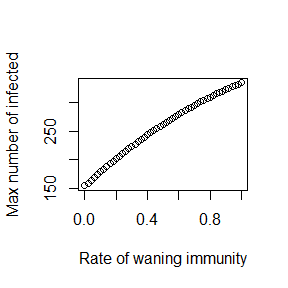# DSAIDE Package Tutorial

## Summary

Dynamical Systems Approaches to Infectious Disease Epidemiology (DSAIDE) is an R package that allows individuals to explore and study concepts of infectious disease epidemiology using dynamical systems models, without the need to read or write computer code. The idea behind the specific structure of the package is that it uses mathematical models to teach infectious disease epidemiology concepts, but does not require the user to write any computer code. Another idea is that if a user wants to continue on their journey of learning modeling and infectious disease epidemiology, they can do so relatively seamlessly with this package by directly accessing and modifying the underlying code. The different use cases for the package are described below.

## Package description

The package consists of several simulations/apps that allow for the simulation and exploration of different topics in infectious disease epidemiology.

The underlying models are written as compartmental dynamical models, either deterministic using differential equations (deSolve package) or stochastic using a Gillespie-type approach (adaptivetau package). A graphical user interface is wrapped around each simulation/app. The graphical user interfaces are written using the functionality of the R Shiny package. This allows exploration of models and infectious disease epidemiology concepts without the need to write any code. At the same time, the package is structured in a modular way that should allow those interested in the actual models and learning R coding to easily move from one stage to another.

Each app is meant to be fully self-explanatory and contains a description of the model, a list of tasks the user could try, and information on further details and readings.

## Intended audience and goal of the package

The audience for this app is individuals interested in infectious disease epidemiology from a systems/modeling perspective. For instance the apps allow exploration of concepts such as patterns of infectious disease incidence, reproductive number concept, extinctions and critical community size, etc. All these concepts are not well described by applying classical epidemiology approaches (i.e. assuming independence between individulas) to infectious diseases, but can readily by understood using a systems/modeling approach.

The documentation for each app tries to be complete and self-contained. However, the information provided inside each app is unlikely sufficient for a complete novice to fully understand the modeling and infectious disease material.

A highly motivated user could likely learn the modeling and infectious disease material covered by the apps on their own by working through some of the references provided for each app and/or general infectious disease modeling textbooks (e.g. (Keeling and Rohani 2008,Vynnycky and White (2010))).

Another intended use is for this package to be part of a course on infectious disease epidemiology/modeling.

## Installing the package

The package can be installed from CRAN or Github. See the documentation on the packages’ Github page for more details.

Package installation is a one-time process, unless R itself is being upgraded/reinstalled. Note that the package depends on other packages, which will also be installed as needed.

## Using the package

The following sections describe the main envisioned ways the content in this R package can be used and extended. The idea is that everyone starts at level 1, and then depending on individual needs and interests, can decide to move on to the next level.

### Level 1: Interactive use through the graphical user interface

The interactive exploration of the models and infectious disease concepts through the graphical user interface is the main intended use of this package. The steps to get there are simple.

#### Load and run the package

Every time a new R/Rstudio session is started, the package needs to be loaded:

library('DSAIDE')

followed by starting the main menu for the package:

dsaidemenu()

This will bring up a graphical menu from which one can select each Shiny app. Each app contains the information needed to understand the underlying model, and has a list of (non exhaustive) suggested tasks to learn about the topic covered by the app. After exploring an app, the user returns to the main menu and eventually exits the main menu and closes the R session. No code needs to be written at this level of exploration and learning.

#### Alternative app use

If for some reason you do not want to go through the main menu, you can call individual apps directly with the function dsaideapps(). By typing

dsaideapps()

you will get a list of available apps. To start a specific app, supply its name in quotation marks to this function, e.g. if you wanted to call the ID control app, you’d call

dsaideapps('IDControl')

Once you exit the app, you are back at the R console and can call the next app.

### Level 2: Directly interacting with the simulation functions

The exploration of the models through the graphical interface is the first and main intended use of the package. Once you are comfortable interacting with the models and have a good understanding of the concepts covered by the different apps, it is possible, without too much effort, to interact with the code more directly. This will provide more flexibility but will require writing some code.

To facilitate direct interaction and modification of the underlying simulations, each app is structured in such a way that the underlying model/simulation is a stand-alone function. For some apps, there are multiple underlying functions involved. You can call/use any of these functions directly, without going through the graphical interface. The ‘Further Information’ tab inside each app provides the name of the corresponding underlying function(s).

Consider as example the first app, called “ID Dynamics Intro”. The simulator function for this model is called simulate_introduction.R. After loading the package (if not already loaded) with

## Loading required package: shiny
## Welcome to the DSAIDE package. Type dsaidemenu() to get started.

the user can learn about the inputs and outputs of the function by looking at its documentation

help('simulate_introduction')

The help file explains that one can run the simulation by specifying initial number of susceptibles and infected, the duration for which the simulation should be run, and the infection and recovery parameters. Unless explicitly specified, the models do not have inherent time units. Instead, those are set by the user based on choices for parameters. It is important to ensure that all quantities have time units. For this simulation, tmax, g, and b are expressed in the same time units, e.g. days or months (or the inverse of those units for the rate parameters). Each parameter has some default. The user can modify those default settings. For instance one can call the simulator with the following settings, overwriting the defaults:

result <- simulate_introduction(S0 = 500, I0 = 5, tmax = 100, g = 0.1,  b = 1/2500)

Calling the simulation function execuctes the underlying dynamical model (here a simple 3 compartment ODE model, as described in the “Model” section of the app). The simulation function produces and returns time-series for the dynamics of each of the variables that are tracked. Users can produce their own plots, e.g. plotting susceptible as function of time:

plot(result$ts[ , "Time"],result$ts[ , "S"],xlab='Time',ylab='Number Susceptible',type='l')The ability to call the simulation functions directly instead of going through the graphical interface allows additional exploration of the models. For instance if one wanted to explore the behavior of a model systematically for different values of a given parameter, this would need to be done manually if run through the graphical interface. Calling the function directly allows one to automate this by wrapping the function inside a loop over the parameter of interest, recording some quantity of interest for each run, and report the result at the end. The following is a simple example, showing a loop over different values of the recovery rate and recording the peak of the outbreak each time, with the final result peak of outbreak as function of recovery time shown in a plot:

gvec = seq(0.01,0.3,by=0.01) #values of recovery rate, g, for which to run the simulation
peak = rep(0,length(gvec)) #this will record the peak values for each g
for (n in 1:length(gvec))
{
#call the simulator function with different values of g each time
result <- simulate_introduction(S0 = 500, I0 = 5, tmax = 200, g = gvec[n],  b = 1/2500)
peak[n] <- max(result\$ts[,"I"]) #record max number of infected for each value of g
}
#plot final result
plot(gvec,peak,type='p',xlab='Rate of recovery',ylab='Max number of infected')Thus, you can add your own custom code to the existing simulator functions and with a few lines of extra code analyze and explore many more questions and scenarios than those accessible through the graphical interface. This provides a lot more flexibility, but requires writing some R code to interface with the supplied simulator functions.

### Level 3: Modifying the simulation functions

As a next step, it is possible to not only interact with the simulation functions, but instead directly access the code and modify the underlying simulator functions. To make this easy, copies of all simulator functions are in a subdirectory called simulatorfunctions inside the DSAIDE package folder. Each function in that folder starts with simulate_. The following R code should tell you where on your computer the directory is that contains the simulator functions:

system.file("simulatorfunctions", package = "DSAIDE")

While the functions in this subfolder are not used to run the code in the package, and one could therefore edit them without breaking the package, it is better to copy the whole folder to a new location. This way the chance of accidentally overwriting any modifications you make is reduced.

All simulator functions are (hopefully) well documented. Some basic to intermediate level of R coding experience is likely required to successfully modify the functions. In addition to modifying the simulator function of interest, you will likely also have to write some additional code to interact with your modified function (as described in Level 2).

The following provides a simple example of this process of modifying a simulator function and exploring its results. Assume that we want to modify the simple SIR model encoded in simulate_introduction.R. After finding the file and making a copy (let’s call the modified function mysimulator.R), we can make modifications. Say we want to include waning immunity with recovered returning to the susceptible class at rate w.

We will need to modify the following lines of code:

old:

simulate_introduction <- function(S0 = 1000, I0 = 1, tmax = 300, g = 0.5, b = 1/1000)

new:

mysimulator <- function(S0 = 1000, I0 = 1, tmax = 300, g = 0.5, b = 1/1000, w = 0)

old:

pars = c(b = b, g = g);

new:

pars = c(b = b, g = g, w = w);

old:

dS =  - b * S * I; #susceptibles
dI = b * S * I - g * I; #infected/infectious
dR = g * I; #recovered

new:

dS =  - b * S * I + w * R; #susceptibles
dI = b * S * I - g * I; #infected/infectious
dR = g * I -  w * R; #recovered

We could now for instance explore how different rates of waning immunity impact the maximum peak size over all outbreaks. This requires a slight modification of the code shown above in Level 2 as follows:

source('mysimulator.R') #to initialize the new function - it needs to be in same directory as this code
wvec = seq(0,1,by=0.02) #values of immunity loss rate, w, for which to run the simulation
peak = rep(0,length(wvec)) #this will record the peak values for each g
for (n in 1:length(wvec))
{
result <- mysimulator(S0 = 1000, I0 = 1, tmax = 300, g = 0.5,  b = 1/1000, w = wvec[n])
peak[n] <- max(result[,"I"])
}
plot(wvec,peak,type='p',xlab='Rate of waning immunity',ylab='Max number of infected')## Developing and contributing new apps to the package

The package is built in a way that makes it (hopefully) easy for others to contribute new simulations/apps. To that end, the package contains a subfolder called docsfordevelopers, which provides information on how the apps are structured and how to add new ones. The information is hopefully complete enough to allow fairly easy development and contribution to the package. If you are interested in contributing new apps, I suggest you get in touch with me, either by email or through the github site for the package.

## Further Information

• I published a paper describing the package and how to use it which you can find and read here. Also use this paper if you want to cite the package. Currently the paper is probably a bit more ‘polished’ than the vignette, but in the future the vignette will be more up-to-date.
• The `documentation.md’ file described above contains more information about the package structure.
• For feedback, bug reports etc., file a github issue.
• A ‘companion’ package to this one, called Dynamical Systems Approaches for Immune Respone Modeling (DSAIRM), focuses on models for analyzing with-host models. It has the same structure as DSAIDE. See the DSAIRM Github site for more information.

## Acknowledgements

For a list of individuals who contributed to this package, see the bottom of this page.